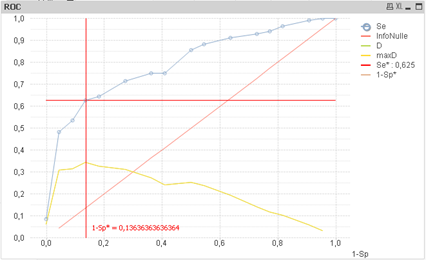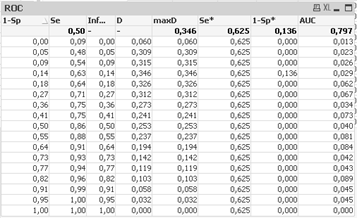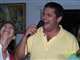# QlikView App Dev

Discussion Board for collaboration related to QlikView App Development.

Announcements
Join “Do More With Qlik” with Mike Tarallo to get a first-hand look at the New Authoring Experience, June 29th: REGISTER HERE
cancel
Showing results for
Did you mean:Contributor II

## Has anyone created an ROC curve in qlikview before? If so, how?

Has anyone created an ROC curve in qlikview before? If so, how?

6 RepliesMVP

When you say ROC, you mean Return on Capital? Although I have not done, but do you have data to try it out?Contributor II
Author

No, ROC stands for Receiver Operating CharacteristicMVP

Hahahahaha, I have no idea what that is... can you share some data and the expected output?Contributor II
Author

So I have field “ABC”, which contains the values 1 – 32. For each number, 1-32, I want to create a point on a chart, with an X and Y value. So the chart will have 32 points, and those points create a line which is an ROC curve. The X and Y values are two different fields, we can call them field X and field Y.Specialist II

Hi,

let's call :

vDiag : variable for the real diagnosis

vMeasure : the measure of the test

in dimension :

create a field (named Threashold) from min to max of variable measure with for example 100 rows :

that says if test considered to be negative or positive

in expression :

TP : true positive :

= sum(if(\$(vMeasure)>Threashold and \$(vDiag) = 1,1,0))

FP : false positive :

= sum(if(\$(vMeasure)>Threashold and \$(vDiag)   = 0,1,0))

TN :true negative

= sum(if(\$(vMeasure)<=Threashold and \$(vDiag)  = 0,1,0))

FN :false negative

= sum(if(\$(vMeasure) <=Threasholdand \$(vDiag)  =1,1,0))

then sensitivity       Se = TP/(TP+FN)

specificity =   Sp = TN/(TN+FP)

1 - Specificity = 1 - TN/(TN+FP)

then export your sheet and reimport to create a new sheet with

Se in Y and 1-Sp in X : blue curveOptimum can be calculated as the maximum distance with the first bissector (y=x)

AUC (Aire Under the Curve) given by :

(if(rowno() = NoOfRows(),1,below([1-Sp]))- [1-Sp]) * (Se + ( if(rowno() = NoOfRows(),1,   below(Se)) - Se)/2)R gives the result : 0.799

regardsPartner

do you have a qvw example with the concept you are explaining? Thanks